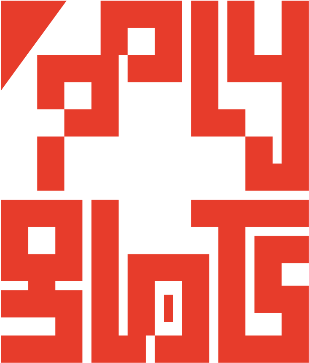# polygl0ts

### EPFL's CTF team# Square CTF 2018 - C10: fixed point

## Description

A handwritten note has been added to C10’s label.

``````If you are a Charvis then this is a simple application of Xkrith’s First and Sixth Theorems.  If you are not a Charvis then you should familiarize yourself with Xkrith’s works.  A primer can be found in Room 100034B.
``````

Note: For the humans out there, this system is built on an oscillator. It’ll disable itself when you find the oscillator’s fixed point.

Good luck!

## Solution

The Javascript code in the given HTML file applies the function below to our input `x`, and gives us the flag if and only if `x` is a fixed point (i.e. `f(x) = x`).

``````function f(x) {
if ((x.substr(0, 2) == '🚀') && (x.slice(-2) == '🚀')) {
return x.slice(2, -2);
}
if (x.substr(0, 2) == '👽') {
return '🚀' + f(x.slice(2));
}
if (x.substr(0, 2) == '📡') {
return f(x.slice(2)).match(/..|/g).reverse().join("");
}
if (x.substr(0, 2) == '🌗') {
return f(x.slice(2)).repeat(5);
}
if (x.substr(0, 2) == '🌓') {
var t = f(x.slice(2));
return t.substr(0, t.length/2);
}

return "";
}
``````

This function reads our input `emoji` by `emoji` interpreting each one of them as an instruction. Those instructions are pushed on the top of a stack. The final output of `f` is the result of applying all those instructions starting from the top of the stack, i.e. last in first out.

The mapping between `emojis` and instructions is the following:

• 👽 -> put 🚀 on the stack
• 📡 -> put `reverse` on the stack
• 🌗 -> put `repeat(5)` on the stack
• 🌓 -> put `substr(0, t.length/2)` on the stack
• 🚀`x`🚀 -> put `x` on the stack (exit case)
• default -> put `''` on the stack (exit case)

We can do the following observations.

1. The only `emoji` that we can create is 🚀.
2. Following from `1.` , the only way we have to “create” other `emojis` is trough the `repeat(5)` operation. Of course the `emojis` we want to “create” have to be in the argument of `repeat(5)`.
3. From what above we conclude that the only acceptable exit case is 🚀`x`🚀, and that `x` has to include all the `emojis` we need to “create”.
4. 📡📡 corresponds to the unit operation.
5. 📡👽📡 translates to `[reverse, 🚀 at beginning, reverse]`. The result of those three instructions will be adding a 🚀 at the end of our current sequence.

Now we have enough information to start building our input!

Let’s define it to be `x`🚀`x`🚀, where `x = y`📡👽📡. This will translate into `[..y.., reverse, 🚀 at beginning, reverse, x]`. Remebering that we have to apply the instructions backwards (i.e. starting from the right), after applying the first four we will end up with the following situation:

• Partial output: `x`🚀
• Stack: `[..y..]` (i.e. the instructions encoded in `y`)

Thus, if we manage to have `y` such that its aggregation corresponds to `repeat(2)` we are done. In other words we want to find a `u`and `v`such that:

``````    1 * 5^u * 2^-v = 2
``````

Unfortunately there is no integer solution to this equation, but we can overcome this limitation by generalizing what stated above. We can define our input to be `x`🚀 repeated `n` times (with `x` defined as before), so that, after applying the first four operations, the situation will be:

• Partial output: `x`🚀 repeated `n-1` times
• Stack: `[..y..]` (i.e. the instructions encoded in `y`)

The equation to solve becomes:

``````    (n-1) * 5^u * 2^-v = n
``````

Which hopefully has `{n = 5, u = 1, v = 2}` as a solution.

We obtain `y=`🌓🌓🌗, thus `x=`🌓🌓🌗📡👽📡. Now we repeat `x`🚀 five times and we are done!

🌓🌓🌗📡👽📡🚀🌓🌓🌗📡👽📡🚀🌓🌓🌗📡👽📡🚀🌓🌓🌗📡👽📡🚀🌓🌓🌗📡👽📡🚀

`flag-2d4584368d09da2187f5`

## Bonus

### Quines

The function `f` of this challenge is actually a quine. Quines are programs that print out their own source code.

### Infinite solutions 📡📡

Since 📡📡 corresponds to the unit operation, there were an infinite number of solutions: one can add to his input as many couples of 📡 as he wants.

Beside this, there are many solutions to the equation presented above and `{n = 5, u = 1, v = 2}` is only one of them.